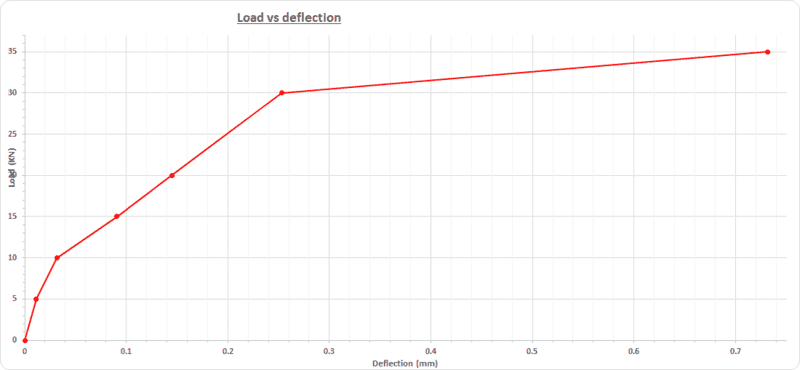# Area Under a Load Vs Deflection Curve

#### tomtomtom1

Hi all

I was wondering if someone could help explain what the area under a load vs deflection curve tells you.

I have a concrete sample which I loaded until it failed.

I plotted the load (kN) and deflection (mm) as shown below.

My question is; if the curve in red can be represented as a function f(x) (which I can do via regression) and I integrated this function from 0 to 0.75 (0.75 was the deflection at failure) then what does this tell me?

What does the area under the curve tell you?From my research the area under a load vs deflection curve is meant to represent energy absorption but others have said it actually represents Work done?

I was wondering if someone could explain?

Thank you.

#### Attachments

• 27.5 KB Views: 202

#### anorlunda

Mentor
Isn't is just a case of units? Work = force * distance. Work is energy. Load is a force. Displacement is a distance. So the area under that curve has units of energy.

#### tomtomtom1

Isn't is just a case of units? Work = force * distance. Work is energy. Load is a force. Displacement is a distance. So the area under that curve has units of energy.
Hi Anorlunda

I know I am being thick but I don't understand.

Are you saying the energy absorption and work are the same thing and if they are then the area under the curve tells you the Work?

Work is defined as "a force is said to do work if, when acting, there is a displacement of the point of application in the direction of the force. For example, when a ball is held above the ground and then dropped, the work done on the ball as it falls is equal to the weight of the ball (a force) multiplied by the distance to the ground (a displacement). Work transfers energy from one place to another, or one form to another. "

Thanks

#### anorlunda

Mentor
Yes, the units of work and the units of energy are the same. When you lift or lower a ball to a different lev then stop, the work you did equals the change in potential energy.

#### CWatters

Homework Helper
Gold Member
If you are bending the beam the area is the work done by you on the beam. If the deformation is elastic then its also the same as the energy stored in the beam when bent. When you allow the beam to bend back straight it does work on you (or you do negative work on the beam).

### The Physics Forums Way

We Value Quality
• Topics based on mainstream science
• Proper English grammar and spelling
We Value Civility
• Positive and compassionate attitudes
• Patience while debating
We Value Productivity
• Disciplined to remain on-topic
• Recognition of own weaknesses
• Solo and co-op problem solving